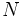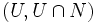# Tame submanifold

Let$M$ be a manifold of dimension$m$ and$N$ a submanifold of dimension$n$. Then$N$ is termed tame in$M$ if for every point$x \in N$, there exists a neighbourhood$U$ of$x$ in$M$ such that the pair$(U, U \cap N)$ is homeomorphic to the pair$(\R^m,\R^n)$ where$\R^n$ is viewed as a linear subspace of$\R^m$.
An example of a submanifold which is not tame is the Alexander horned sphere in$\R^3$.